﻿ IIT JAM Statistics Coaching Delhi | IIT JAM Stat Coaching Mumbai•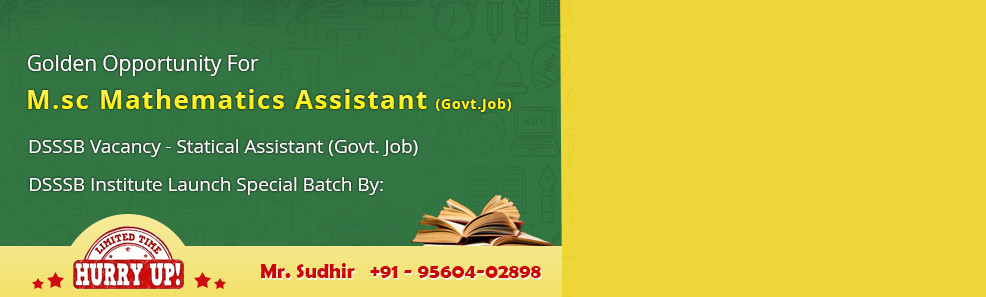•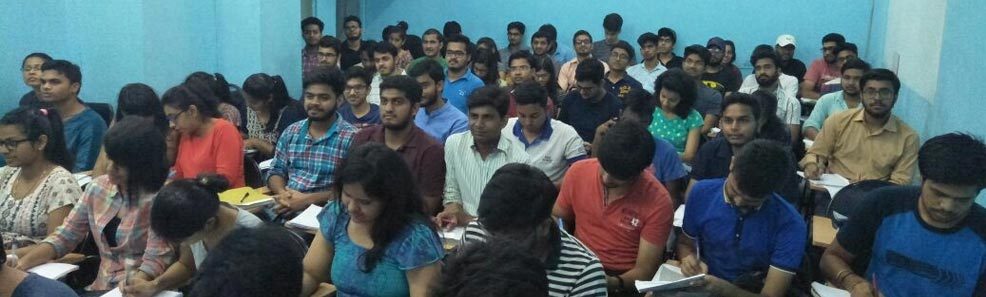•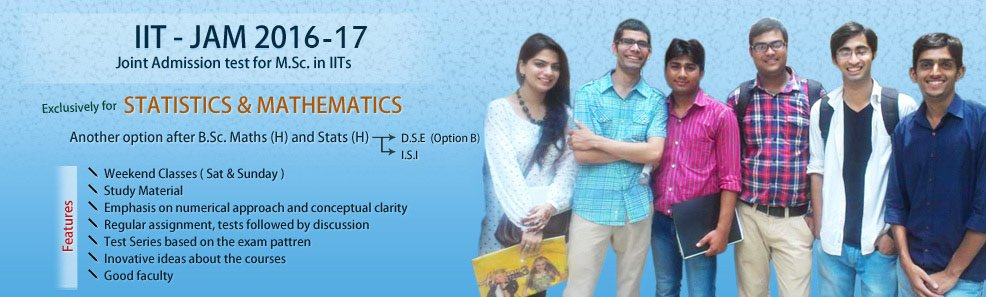•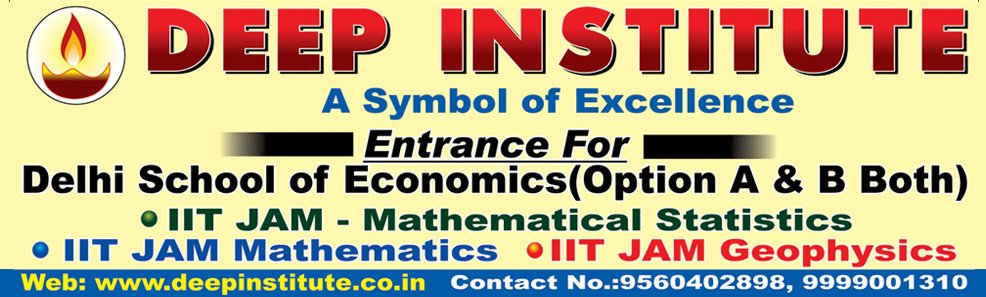•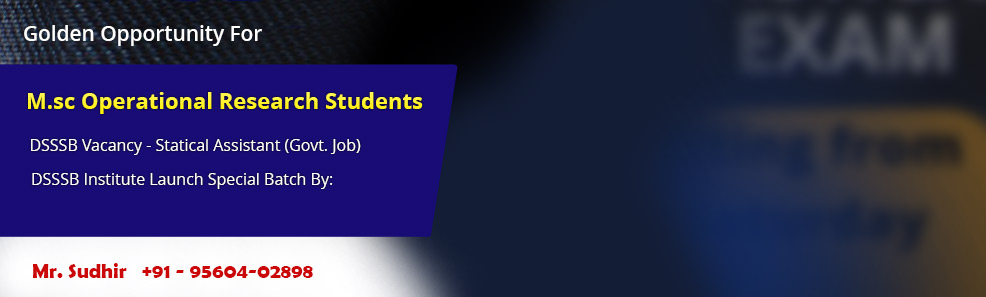•# IIT JAM Statistics

The Mathematical Statistics (MS) test paper of IIT JAM Entrance Examination contains questions from both Maths and Statistics subjects. The question paper comprises of Mathematics (40% weightage) and Statistics (60% weightage). Deep Institute not only provides coaching and traning for the subject but also give study material (Researched and Developed by Our Experts) to all the students. Sample test papers are also available at the institute for everyone to do the practise.

Call us to join IIT JAM Statistics coaching classes in Delhi, IIT JAM Statistics coaching classes in Mumbai and shape your future.

## Mathematical Statistics Syllabus

Mathematics:

Sequences and Series: Convergence of sequences of real numbers, Comparison, root and ratio tests for convergence of series of real numbers.

Differential Calculus: Limits, continuity and differentiability of functions of one and two variables. Rolle's theorem, mean value theorems, Taylor's theorem, indeterminate forms, maxima and minima of functions of one and two variables.

Integral Calculus: Fundamental theorems of integral calculus. Double and triple integrals, applications of definite integrals, arc lengths, areas and volumes.

Matrices: Rank, inverse of a matrix. systems of linear equations. Linear transformations, eigenvalues and eigenvectors. Cayley-Hamilton theorem, symmetric, skew-symmetric and orthogonal matrices.

Differential Equations: Ordinary differential equations of the first order of the form y' = f(x,y). Linear differential equations of the second order with constant coefficients.

Statistics:

Probability: Axiomatic definition of probability and properties, conditional probability, multiplication rule. Theorem of total probability. Bayes' theorem and independence of events.

Random Variables: Probability mass function, probability density function and cumulative distribution functions, distribution of a function of a random variable. Mathematical expectation, moments and moment generating function. Chebyshev's inequality.

Standard Distributions: Binomial, negative binomial, geometric, Poisson, hypergeometric, uniform, exponential, gamma, beta and normal distributions. Poisson and normal approximations of a binomial distribution.

Joint Distributions: Joint, marginal and conditional distributions. Distribution of functions of random variables. Product moments, correlation, simple linear regression. Independence of random variables.

Sampling distributions: Chi-square, t and F distributions, and their properties.

Limit Theorems: Weak law of large numbers. Central limit theorem (i.i.d.with finite variance case only).

Estimation: Unbiasedness, consistency and efficiency of estimators, method of moments and method of maximum likelihood. Sufficiency, factorization theorem. Completeness, Rao-Blackwell and Lehmann-Scheffe theorems, uniformly minimum variance unbiased estimators. Rao-Cramer inequality. Confidence intervals for the parameters of univariate normal, two independent normal, and one parameter exponential distributions.

Testing of Hypotheses: Basic concepts, applications of Neyman-Pearson Lemma for testing simple and composite hypotheses. Likelihood ratio tests for parameters of univariate normal distribution.

Books :-
1. Real Analysis ( N.P BALI)
2. DIFFERENTIAL CALCULUS (SHANTI NARAIN)
3. Integral calculus (SHANTI NARAIN) OR CALCULUS BY STRAUSS
4. MATRICES BY S.C GUPTA OR BY A.R VASISTHA
5. Differential Equation by G.F SIMMONS
6. FUNDAMENTAL OF MATHEMATICAL STATISTICS BY S.C GUPTA &V.K KAPUR
7. Estimation theory by BANSILAL